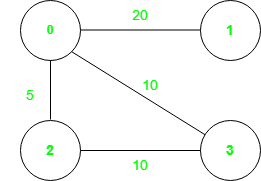# Check if an edge is a part of any Minimum Spanning Tree

• Last Updated : 29 Dec, 2021

Given a connected undirected weighted graph in the form of a 2D array where each row is of the type [start node, end node, weight] describing an edge, and also two integers (A, B). Return if the edge formed between (A, B) is a part of any of the Minimum Spanning Tree (MST) of the graph.

Minimum Spanning Tree (MST): This is a special subgraph of the graph, such that each and every vertex is connected and the overall sum of the weights of the edges of this subgraph is as minimum as possible. A graph can have multiple minimum spanning trees.

Examples:

Input: graph = [[0 ,1, 20] , [0 , 2 , 5] , [ 0, 3, 10 ] , [ 2, 3, 10]], A = 2, B = 3
Output:  True
Explanation : 2 minimum spanning trees with can be generated which will have weight 35. The connections of the trees are
1st: [ (0,1) , (0,3) , (0,2)] => 20 + 10 + 5 = 35
2nd: [ ( 0 , 1) , ( 0 , 2 ) , ( 2 , 3) ] => 20 + 5 + 10 = 35
As it can be seen , the edge ( 2, 3) is present in second MST.

The graph is shown in image:Input: graph = [[0 ,1, 20] , [0 , 2 , 5] , [ 0, 3, 10 ] , [ 2, 3, 20]], A = 2, B = 3
Output: False
Explanation: Only 1 minimum spanning trees with weight 35 can be generated,
but edge (2, 3) is not included.
[(0,1) , (0,3) , (0,2)] => 20 + 10 + 5 = 35

The graph is given in the imageApproach : Kruskal Algorithm and Prim’s Algorithm are the two most used algorithms that can be used to find MST of any graph. In this article, the solution is based on the Kruskal algorithm. Follow the steps mentioned below to solve the problem using this approach:

1. Find the minimum spanning tree cost of the entire graph, using the Kruskal algorithm.
2. As the inclusion of the edge (A, B) in the MST is being checked, include this edge first in the minimum spanning tree and then include other edges subsequently.
3. Finally check if the cost is the same for both the spanning trees including the edge(A, B) and the calculated weight of the MST.
4. If cost is the same, then edge (A, B) is a part of some MST of the graph otherwise it is not.

Below is the implementation of the above approach:

## Python3

 `# Python program to implement above approach`` ` `# Class to implement disjoint set union``class` `dsu:``    ``def` `__init__(``self``):``        ``self``.parent ``=` `{}``        ``self``.rank ``=` `{}`` ` `    ``# Function to find parent of a node``    ``def` `find(``self``, x):``        ``if` `(x ``not` `in` `self``.parent):``            ``self``.rank[x] ``=` `1``            ``self``.parent[x] ``=` `x``        ``if` `(``self``.parent[x] !``=` `x):``            ``self``.parent[x] ``=` `\``                ``self``.find(``self``.parent[x])``        ``return` `(``self``.parent[x])`` ` `    ``# Function to perform union``    ``def` `union(``self``, u, v):``        ``p1 ``=` `self``.find(u)``        ``p2 ``=` `self``.find(v)`` ` `        ``# If do not belong to same set``        ``if` `(p1 !``=` `p2):``            ``if` `(``self``.rank[p1]``                    ``< ``self``.rank[p2]):``                ``self``.parent[p1] ``=` `p2`` ` `            ``elif``(``self``.rank[p1]``                 ``> ``self``.rank[p1]):``                ``self``.parent[p2] ``=` `p1``            ``else``:``                ``self``.parent[p2] ``=` `p1``                ``self``.rank[p1] ``+``=` `1``            ``return` `(``True``)`` ` `        ``# Belong to same set``        ``else``:``            ``return` `False`` ` ` ` `class` `Solution:`` ` `    ``# Find the MST weight``    ``def` `kruskal(``self``, include, edges, a, b):``        ``obj ``=` `dsu()``        ``total ``=` `0`` ` `        ``# If include is True , then include``        ``# edge (a,b) first``        ``if` `(include):``            ``for` `(u, v, wt) ``in` `edges:`` ` `                ``# As graph is undirected so``                ``# (a,b) or (b,a) is same``                ``# If found break the for loop``                ``if` `(u, v) ``=``=` `(a, b) ``or` `\``                        ``(b, a) ``=``=` `(u, v):``                    ``val ``=` `obj.union(a, b)``                    ``total ``+``=` `wt``                    ``break`` ` `        ``# Go on adding edge to the disjoint set``        ``for` `(u, v, wt) ``in` `edges:`` ` `            ``# Nodes (u,v) not belong to``            ``# same set include it``            ``if` `(obj.union(u, v)):``                ``total ``+``=` `wt`` ` `        ``# Finally return total weight of MST``        ``return` `(total)`` ` `    ``# Function to find if edge (a, b)``    ``# is part of any MST``    ``def` `solve(``self``, edges, a, b):`` ` `        ``# Sort edges according to weight``        ``# in ascending order``        ``edges.sort(key``=``lambda` `it: it[``2``])`` ` `        ``# Not included edge (a,b)``        ``overall ``=` `self``.kruskal(``False``,``                               ``edges, a, b)`` ` `        ``# Find mst with edge (a,b) included``        ``inc ``=` `self``.kruskal(``True``,``                           ``edges, a, b)`` ` `        ``# Finally return True if same``        ``# else False``        ``if` `(inc ``=``=` `overall):``            ``return` `(``True``)``        ``else``:``            ``return` `(``False``)`` ` ` ` `# Driver code``if` `__name__ ``=``=` `"__main__"``:``    ``obj ``=` `Solution()``    ``graph ``=` `[[``0``, ``1``, ``20``], [``0``, ``2``, ``5``],``             ``[``0``, ``3``, ``10``], [``2``, ``3``, ``10``]]``    ``A, B ``=` `2``, ``3``    ``val ``=` `obj.solve(graph, A, B)``    ``if` `(val):``        ``print``(``"True"``)``    ``else``:``        ``print``(``"False"``)`
Output
```True
```

Time Complexity: O(E * logV). where E is the number of edges and V is the number of vertices.
Auxiliary Space: O(V)

My Personal Notes arrow_drop_up